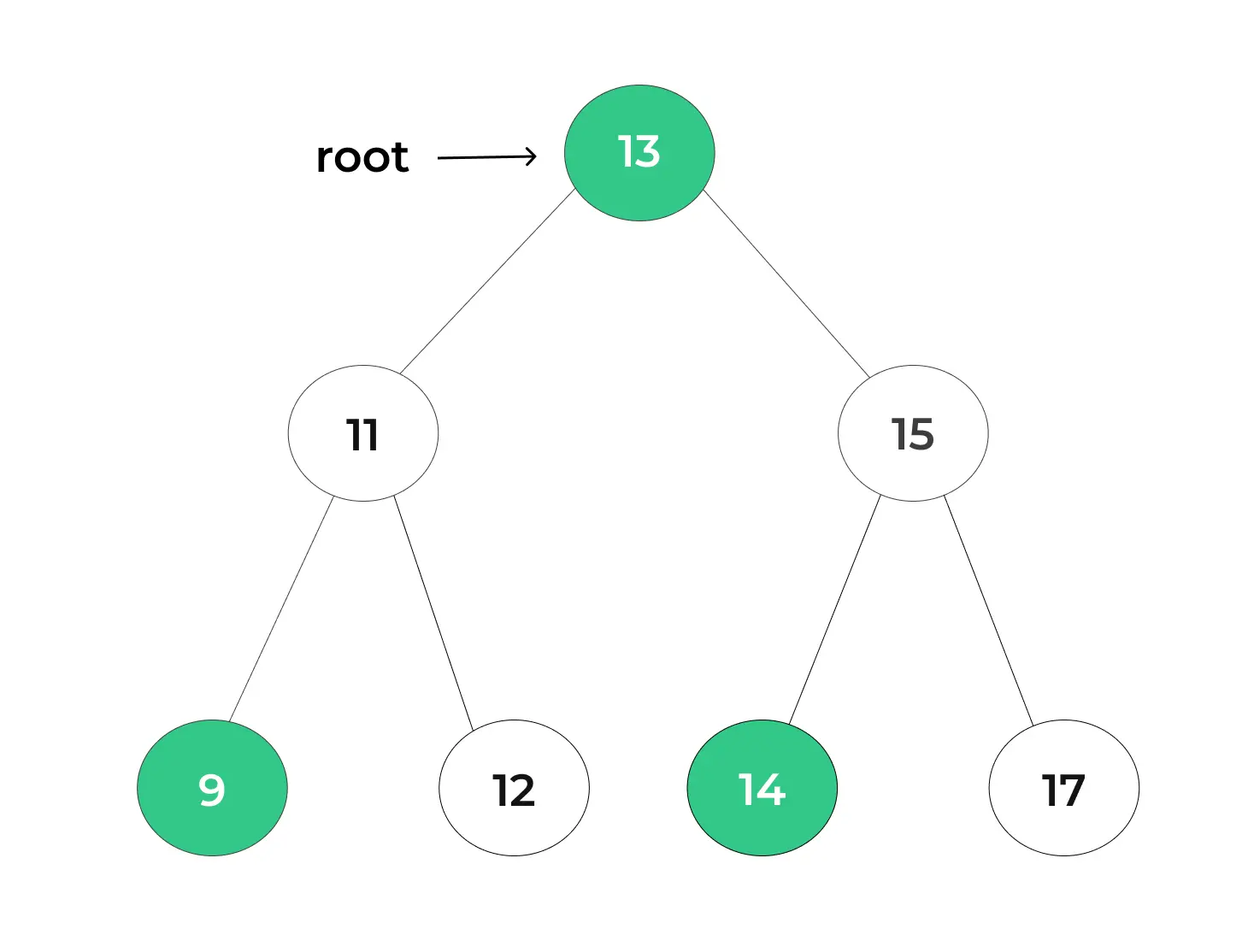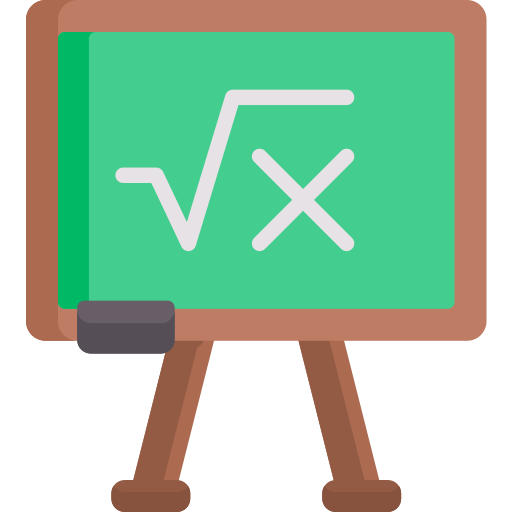# Kth Largest Element in a BST

Largest## Introduction

When it comes to efficient data retrieval in Binary Search Trees (BSTs), finding the Kth largest element is a valuable operation. This page delves into the techniques and strategies used to identify the Kth largest element within a BST.

Let us explore two approach for finding kth largest element in BST i.e. Recursive and Iterative.

## Understanding the K'th Smallest Element in BST

To find the Kth largest element in a BST, you need to traverse the tree in a way that allows you to visit nodes in descending order of their values. This often involves a reverse inorder traversal, starting from the right subtree and moving towards the left subtree.

## What is Kth Largest Element?

The Kth largest element in a dataset or collection is the element that has the Kth highest value when the elements are sorted in descending order. In the context of a Binary Search Tree (BST), it refers to finding the Kth largest element among the values stored in the tree.

For example, in the dataset [5, 8, 2, 10, 1, 7, 9], the 3rd largest element is 7 because, when sorted in descending order, it occupies the 3rd position.

In a BST, the Kth largest element can be found by performing a reverse inorder traversal, which explores the tree nodes in descending order of their values. This operation is often used in data analysis, sorting algorithms, and percentile calculations.

## Example :

Given a Binary Search Tree (BST) with its root node and an integer K as input, the task is to determine the Kth largest element in the BST.

• For instance, consider the following BST: if K equals 3, the result would be 14, and if K is set to 5, the output would be 12.## Approaches to Find Kth Largest Element in BST

There are multiple approaches to find the Kth largest element in a BST, and they primarily differ in their traversal methods. The two most commonly used approaches are:

1. Reverse Inorder Traversal (Recursive): In this approach, you perform a recursive reverse inorder traversal of the BST. As you traverse the tree, you maintain a count of visited nodes. When the count reaches K, you have found the Kth largest element.

2. Reverse Inorder Traversal (Iterative): Similar to the recursive approach, this method employs an iterative reverse inorder traversal using a stack to mimic the call stack of a recursive function. It’s a memory-efficient alternative.

## Choosing the Right Approach

The choice between recursive and iterative approaches often depends on your specific requirements. Recursive methods may be more intuitive to implement, while iterative methods may offer better memory efficiency in terms of space complexity.

## Code for Kth Largest Element in BST

### 1. UsingRecursive Approach :

Here’s a code snippet :

```class TreeNode:
def __init__(self, val=0, left=None, right=None):
self.val = val
self.left = left
self.right = right

def kth_largest_recursive(root, k):
def reverse_inorder_traversal(node):
nonlocal k, result
if not node or k == 0:
return

# Traverse the right subtree first
reverse_inorder_traversal(node.right)

# Process the current node
k -= 1
if k == 0:
result = node.val
return

# Traverse the left subtree
reverse_inorder_traversal(node.left)

result = None
reverse_inorder_traversal(root)
return result

# Example usage:
if __name__ == "__main__":
root = TreeNode(7)
root.left = TreeNode(3)
root.right = TreeNode(10)
root.left.left = TreeNode(1)
root.left.right = TreeNode(5)

k = 4

result_recursive = kth_largest_recursive(root, k)
print("The", k, "th largest element (recursive approach) is", result_recursive)
```

### Output :

```The 4th largest element is 3.
```

### Explanation :

• The recursive approach performs a reverse inorder traversal, counting nodes until it reaches the Kth largest element.
• For the given BST, the output for K = 3 is the same for both approaches, correctly identifying the 3rd largest element, which is 4. These methods efficiently find the Kth largest element, making them versatile tools for data analysis and search algorithms.

### 2. Using Iterative Approach :

Let’s see the code.

```class TreeNode:
def __init__(self, val=0, left=None, right=None):
self.val = val
self.left = left
self.right = right

def kth_largest_iterative(root, k):
stack = []
current = root

while True:
while current:
stack.append(current)
current = current.right

current = stack.pop()
k -= 1

if k == 0:
return current.val

current = current.left

# Example usage:
if __name__ == "__main__":
root = TreeNode(7)
root.left = TreeNode(3)
root.right = TreeNode(10)
root.left.left = TreeNode(1)
root.left.right = TreeNode(5)

k = 4

result_iterative = kth_largest_iterative(root, k)
print("The", k, "th largest element (iterative approach) is", result_iterative)
```

### Output :

```The 4th largest element is 3.
```

### Explanation :

• The iterative approach uses a stack to simulate this traversal.
• For the given BST, the output for K = 3 is the same for both approaches, correctly identifying the 3rd largest element, which is 4. These methods efficiently find the Kth largest element, making them versatile tools for data analysis and search algorithms.

## Significance of Finding Kth Largest Element in BST

Finding the Kth largest element in a Binary Search Tree (BST) holds significant importance in various applications and scenarios. Here are some of the key reasons why this operation is valuable:

1. Data Analysis: In data analysis and statistics, finding the Kth largest element allows for calculating percentiles and quartiles. It helps in understanding data distributions and identifying values that fall within specific percentiles.

2. Outlier Detection: Identifying the Kth largest element can assist in outlier detection. Outliers are data points that significantly deviate from the norm, and knowing the Kth largest value helps in spotting these anomalies.

3. Sorting Algorithms: Finding the Kth largest element is a fundamental step in various sorting algorithms, such as quicksort and heapsort. It plays a pivotal role in partitioning data efficiently.

## Complexity analysis for Kth Largest Element in BST

The complexity analysis for finding the Kth largest element in a Binary Search Tree (BST) depends on the chosen approach. Let’s analyze the time and space complexities for both recursive and iterative methods:

##### Recursive Approach:
• Time Complexity:

• In the worst case, the recursive approach performs a reverse inorder traversal of the entire BST.
• The time complexity is O(N), where N is the number of nodes in the tree.
• However, in many cases, especially in well-balanced trees, the average time complexity is O(log N), as the traversal only visits a subset of nodes.
• Space Complexity:

• The space complexity is determined by the recursion stack.
• In the worst case, when the tree is skewed (completely unbalanced), the space complexity is O(N), as the stack depth equals the number of nodes.
• In balanced trees, the space complexity is O(log N), as the stack depth is limited by the tree height.
##### Iterative Approach:
• Time Complexity:

• The iterative approach also performs a reverse inorder traversal but without recursion.
• In the worst case, it visits all nodes once.
• The time complexity is O(N), where N is the number of nodes in the tree.
• Space Complexity:

• The space complexity is determined by the stack used for iteration.
• In the worst case, it can be O(N) when the tree is skewed.
• In balanced trees, it is O(log N) due to the limited stack depth.
##### To wrap it up:

In summary, finding the Kth largest element in a BST is a valuable operation that can be efficiently accomplished using various traversal techniques. Understanding the principles of BSTs and these approaches equips you with the skills to tackle this task in diverse real-world scenarios.

Whether you’re working with large datasets, implementing search algorithms, or analyzing data distributions, the ability to find the Kth largest element empowers you to make informed decisions and gain insights from your data.

### Related Banners

Get PrepInsta Prime & get Access to all 200+ courses offered by PrepInsta in One SubscriptionQuestion 1.

In what applications is finding the Kth largest element useful?

Finding the Kth largest element is valuable in data analysis, outlier detection, sorting algorithms, and optimizing database queries. It helps in percentile calculations and decision-making based on ordered data.Question 2.

Is there a difference in efficiency between recursive and iterative approaches?

In terms of time complexity, both recursive and iterative approaches have the same worst-case time complexity. However, the iterative approach can be more memory-efficient as it doesn’t use the call stack for recursion.Question 3.

Can I use the same algorithm for finding the Kth smallest element in a BST?

Yes, the same algorithm used to find the Kth largest element can be modified slightly to find the Kth smallest element in a BST. Instead of a reverse inorder traversal, perform a regular inorder traversal and count nodes until you reach the Kth smallest element.

## Get over 200+ course One Subscription

Courses like AI/ML, Cloud Computing, Ethical Hacking, C, C++, Java, Python, DSA (All Languages), Competitive Coding (All Languages), TCS, Infosys, Wipro, Amazon, DBMS, SQL and others